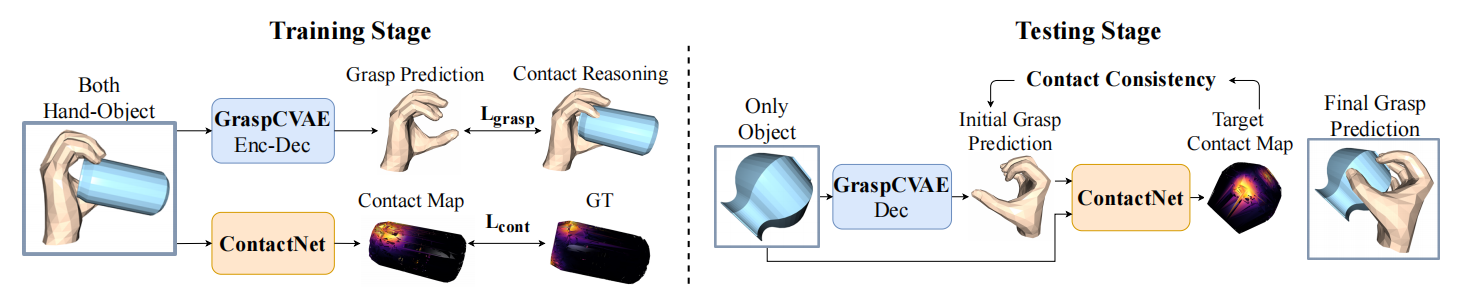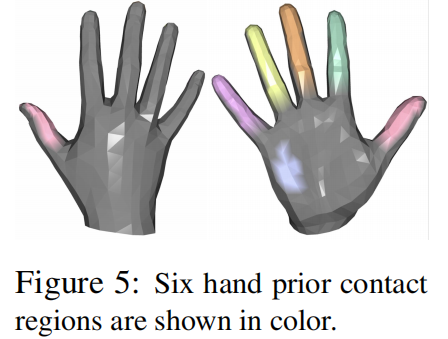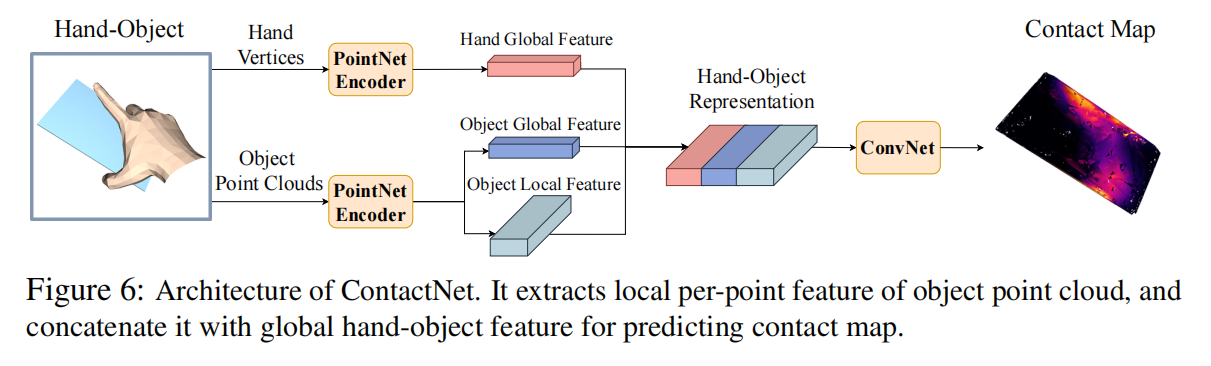# (ICCV2021)Hand-Object Contact Consistency Reasoning for Human Grasps Generation

​ 这个工作的目标是给定一个待抓取物体的点云，创建出对应的抓取手型网格。创建出的hand mesh首先得是自然、真实的，其次需要物理上能够抓紧物体。确保物体和合成的手型之间的合理的接触是得到 high-quality 和 stable 的 grasp的关键。

​ 为了解决这个问题，我们利用手和物体的接触信息，并且确保它们是一致的。我们提出了两个网络，一个是生成式的 GraspCVAE来合成 grasping hand mesh，一个判断式的网络 ContactNet 来对接触区域建模。### 训练阶段

​ 两个网络分别训练。一个学习创建grasp，一个预测object的contact map。对于GraspCVAE来说，要同时输入手型和物体点云，来合成 hand reconstruction paradiam。为了训练Grasp CVAE，我们提出了两种loss来保证 hand-object consistency：一个强制先验的 hand contact的顶点接近物体表面，另一个loss是促进object的contact region被手接触到的。

### 测试阶段

​ 给定一个待测试的物体，首先我们通过 GraspCVAE decoder 来创建出初始的抓型。创建出的抓型和物体点云一起传进 ContactNet 中来预测目标的 contact map。因为此时 ContactNet 已经用GT训练完成了，且其 Loss的目标是 penetration-free、且手指紧贴物体，所以我们认为此时 ContactNet 预测出来的 contact map是理想的接触。 我们使用 ContactNet 预测出来的 contact map作为 GT，来继续优化我们 GraspCVAE 创建出来的 initial grasp。如果GraspCVAE所创建出来的抓型是比较完美的，那么它的抓型直接计算的 contact map应该和 ContactNet 预测出来的 contact map一致。我们使用这个一致性作为自监督的signal来在测试阶段优化 GraspCVAE创建出来的 grasps。

### 训练过程

​ 在训练过程中，手是通过点云$P^h\in R^{778\times 3}$来建模的，物体是通过点云$P^o\in R^{N\times 3}$来建模的。我们分别使用2个PointNet来提取特征$F^h, F^o\in R^{1024}$。然后把两个特征接在一起传入 Encoder中，输出$\mu \in R^{64}, \sigma^2\in R^{64}$。我们从高斯分布中采样隐变量$z$。

​ Decoder输入是隐变量$z$和物体的特征$F^{o}$来重建 hand mesh。Hand mesh是通过可微分的 MANO 模型表示的。MANO模型通过形状参数$\beta \in R^{10}$来描述人的不同的手型，而$\theta \in R^{51}$ 来描述关节的旋转角和基关节的平移。给定 Decoder 预测出来的参数$(\hat{\beta},\hat{\theta})$，MANO模型可以创建出一个可微分层来创建出手的模型，即$\hat{M}=(\hat{V}, \hat{F})$，其中$\hat{V}\in R^{778\times 3}$代表网格的顶点，而$\hat{F}$ 代表 mesh 的面。

### Object-centric Loss

​ 当我们关注被抓取物体的时候，它上面总有一些区域是和手接触的。我们希望我们的手和这些区域是尽可能近的，所以就有了 Object-centric Loss 。具体地来说，从GT的 hand-object interaction中，我们可以计算出 object contact map $\Omega\in R^N$，具体计算流程如下：

​ 我们计算每个物体点到达最近的 hand vertex的距离，记为$D(P^o)$，并且归一化，记为$\Omega = f(D(P^o))$，且有
$$f(x)=1-2(\text{Sigmoid}(2x) - 0.5)$$​ 如上图所示，每个点都被赋予了一个0~1的值，作为我们的 Contact Map。归一化的目的是让网络关注那些接近 hand 的区域。这样我们就可以使用如下 Loss 来达到我们的目的：
$$L_O=||\hat{\Omega}-\Omega||_2^2$$

### Hand-centric Loss

​ 我们预先定义好了手上的一些接触顶点 $V^p$，如下图所示：​ 给定hand contact vertices 的预测位置，我们认为每个手上 contact region vertex附近的物体点是一些潜在的接触点。对于物体点云上的每个点$P_i^o$，我们计算它到最近的接触顶点的距离，如果小于一个阈值，我们就认为它是物体上的接触点。我们 Hand-centric loss的目标就是让手上的接触顶点尽可能靠近物体：
$$L_H=\sum_iD(P^o_i), \text{for all } D(P^o_i\leq \tau)$$
​ 我们定义$\tau=1\text{cm}$，最终我们的loss的组合如下：
$$L_{grasp}=L_{baseline}+\lambda_OL_O+\lambda_HL_H$$
​ 简单的来说$L_O$回答了哪里是可以抓取的位置的问题，而$L_H$回答了哪些手指应当接触的问题。

## ContactNet

​ 输入手点云$P^h\in R^{778\times 3}$和物体点云$P^o\in R^{N\times 3}$。网络的目标是预测出 contact map $\Omega^c\in R^N$。​ 网络的Loss设定为预测出的 contact map 和 GT 的 $L_2$范数，即
$$L_{cont}=||\Omega^c-\Omega||_2^2$$

​ 在测试过程中，对于一个物体点云，我们先使用 CVAE 创建出一个手的mesh $\hat{M}$，如下图所示：​ 我们可以通过$\hat{M}$ 手动计算出对应的 contact map $\Omega_{\hat{M}}$。然后我们继续把物体点云和 $\hat{M}$ 传入到 ContactNet 中得到较好的 $\Omega^c$。我们希望让我们的$\Omega_{\hat{M}}$尽可能接近$\Omega^c$，所以这是一种自监督的方式继续优化GraspCVAE的参数，Loss如下：
$$L_{TTA}=L_{refine}+\lambda_HL_H+\lambda_pL_{penetration}$$
​ 我们使用这个Loss来更新 GraspCVAE decoder的参数，冻结两个网络的其余部分。

## 实验部分和代码部分

​ 此处有需要再看。需要关注的是手和物体的数据集，如Obman Dataset。以及手的建模的MANO模型的细节，以及一些度量指标。还有4种TTA的具体逻辑和比较实验，TTA是一个比较新颖的逻辑，有时候看完TTA并不知道它能不能work，还是要加深这部分的理解。

(ICCV2021)Hand-Object Contact Consistency Reasoning for Human Grasps Generation

https://kami-code.com/2022/03/13/GraspTTA/

Kami-code

2022-03-13

2022-03-16